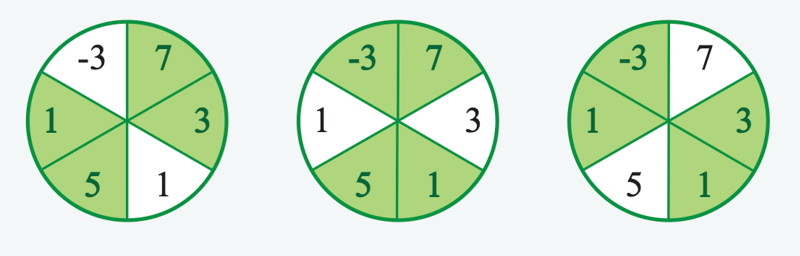Amazon Online Assessment 2021 (OA) - Efficient Harvest

A farmer uses pivot irrigation to water a circular field of crops. Due to varying conditions, the field does not produce consistently. The farmer wants to achieve maximum profit using limited resources for harvest. The field is segmented into a number of equal segments, and a profit is calculated for each segment. This profit is the cost to harvest versus the sale price a the produce. The farmer will harvest a number of contiguous segments along with those opposite. Determine the maximum profit the farmer can achieve.For example, the product list Is divided into n = 6 sections and will select k = 2 contiguous sections and those opposite to invest in. The profit estimates are profit = [1, 5, 1, 3, 7, -3] respectively. The diagrams below show the possible choices with profits at the 9 o'clock position and filling counterclockwise.

The profit levels, from left to right, are 1+5+7+3=16, 5+1+7+-3=10, and 1+3+-3+1=2. The maximum profit is 16.

Input

• k: an integer that denotes half of the needed number of products within the list
• profit: an array of integers that denote the profit from investing in each of the products

Output

the maximum profit possible

Examples

Example 1:

Input:

1k = 2
2profit = [1, 5, 1, 3, 7 -3]

Output: 16

Explanation:

The profit levels, from left to right, are 1+5+7+3=16, 5+1+7+-3=10, and 1+3+-3+1=2. The maximum profit is 16.

Example 2:

Input:

1k = 1
2profit = 3 -5

Output: -2

Explanation:

Here k=1 and n=2. The seller will choose 2*k=2 products. In this case, it is the entire list, so overall profit is 3+-5=-2.

Constraints

• 1 <= k <= n/2
• 2 <= n <= 10^5
• n is even
• 0 <= |profit[i]| <= 10^9

Title

Script

Lorem Ipsum is simply dummy text of the printing and typesetting industry. Lorem Ipsum has been the industry's standard dummy text ever since the 1500s, when an unknown printer took a galley of type and scrambled it to make a type specimen book.

Contrary to popular belief, Lorem Ipsum is not simply random text.

1  >>> a = [1, 2, 3]
2  >>> a[-1]
3  3